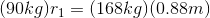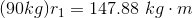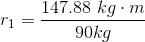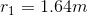# High School Physics : Understanding Torque

## Example Questions

### Example Question #1 : Understanding Torque

A child spins a top with a radius of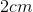with a force of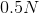. How much torque is generated at the edge of the top?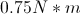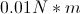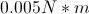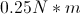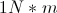Explanation:

Torque is a force times the radius of the circle, given by the formula: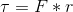In this case, we are given the radius in centimeters, so be sure to convert to meters: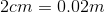Use this radius and the given force to solve for the torque.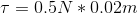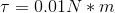### Example Question #2 : Understanding Torque

Two children are trying to balance on a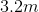see-saw. One child has a mass of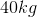the other has a mass of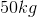. If the see-saw is balanced perfectly in the middle and thechild is sitting at one end of the see-saw, how far from the center should thechild sit so that the system is perfectly balanced?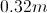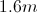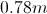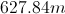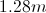Explanation:

If the see-saw isin total, then it has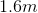on either side of the fulcrum.

The question is asking us to find the equilibrium point; that means we want the net torque to equal zero.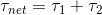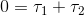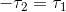Now, find the torque for the first child.We are going to use the force of gravity for the force of the child.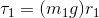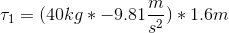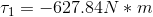When thinking of torque, treat the positive/negative as being clockwise vs. counter-clockwise instead of up vs. down. In this case, child one is generating counter-clockwise torque. That means that since, child two will be generating clockwise torque.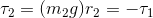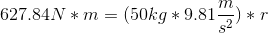Solve for the radius (distance) of the second child.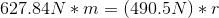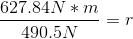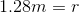### Example Question #3 : Understanding Torque

A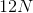force is applied perpendicularly to a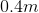wrench. How much torque is generated?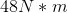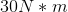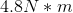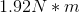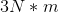Explanation:

The formula for torque is: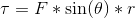In this formula,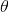is the angle the force makes with the lever arm. Since our force is applied perpendicularly, this angle will be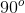. Use this angle, the force applied, and the length of the lever arm to calculate the torque.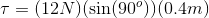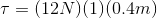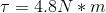### Example Question #4 : Understanding Torque

In ancient Greece, construction workers would use long levers to lift up boulders. Assume a boulder has a mass of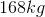and a certain construction worker has a mass of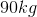. The pivot point of the lever is placed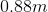from the boulder and the construction worker stands on the opposite end. How long does the lever need to be between the pivot point and the construction worker in order for the system to be in equilibrium?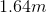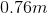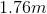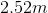Explanation:

We know that the system will be in equilibrium at the final point, meaning that the final net torque must be zero. That means that the torque generated by the boulder and the torque generated by the construction worker should be equal.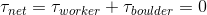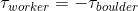The negative sign is due to the placement of the two masses on opposite sides of the fulcrum. Essentially, one mass will have a positive radius and the other will have a negative radius. Use the definition of torque to expand this equation.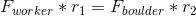In this case, the forces will be the weights of the two objects due to gravity.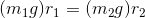We can cancel the acceleration due to gravity from the equation to simplify.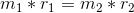Using the given information from the question, we can solve for the distance between the worker and the fulcrum. We know the mass of the worker, the mass of the boulder, and the distance between the boulder and the fulcrum.